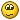## Reply: Estimate Activity Durations - Activity Variance

 Name E-mail Your e-mail address will never be displayed on the site. Subject Message [quote="Khurram Hussain" post=3045]Dear Stefaan: Good day! To calculate the standard deviation, you would calculate the variance and then square root it. The first statistical argument of having a variance is that we need to calculate the standard deviation. Variance is the statistical measure of calculating the dispersion of the data. However since variance is in the squared units, it doesn’t make much sense when using the measure practically. Hence we square root it to get a measure in the same units as of the data. For example variance of a sample of lengths measured in meters will be in squared-meters; however the standard deviation will be in meters which make more sense and helps in calculating the ranges. Variance is calculated by computing squared deviations (from the mean) for every observation and adding them up together. If the deviations were not squared, the result would have been zero. However, since this process results in measurements in squared-units, we take a square root of it to bring it back to the original units. For a detailed debate, you need to see text on statistics. If you don't want to go deep in statistics, then please remember that calculating variances is a statistical obligation. Regards, Khurram[/quote]

## Topic History of : Estimate Activity Durations - Activity Variance

Max. showing the last 6 posts - (Last post first)
3 years 2 months ago #7373

ShervinHey Everybody... I find myself asking the same question as Stefaan above. What is the purpose of the Activity Duration Variance and where is it put to use?

Is it only used in calculating the total project duration variance? Is it fair to say that it's the sum of the absolute values of all activity Standard deviations?

Khurram Hussain's explanation goes over how you calculate the value. What I want to know is where it fits in! What can you do with it that you can't do with adding up the absolute value of all SDs to arrive at the project duration range?

6 years 6 months ago #3046

Stefaan DehertefeltHi Khurram,

Thank you very much for the explanation, it makes sense to me.
For the moment I would not like to go too much into details on the statistical theory.

For the PMP exam I will just remember that:
1. The activity Variance will give you the expected variance in the activity's duration.
2. The standard deviation for a given series of activities is calculated by taking the square root of the sum of the activity variances

Thanks again,
Stefaan
6 years 6 months ago #3045Dear Stefaan:

Good day!

To calculate the standard deviation, you would calculate the variance and then square root it. The first statistical argument of having a variance is that we need to calculate the standard deviation.

Variance is the statistical measure of calculating the dispersion of the data. However since variance is in the squared units, it doesn’t make much sense when using the measure practically. Hence we square root it to get a measure in the same units as of the data. For example variance of a sample of lengths measured in meters will be in squared-meters; however the standard deviation will be in meters which make more sense and helps in calculating the ranges.

Variance is calculated by computing squared deviations (from the mean) for every observation and adding them up together. If the deviations were not squared, the result would have been zero. However, since this process results in measurements in squared-units, we take a square root of it to bring it back to the original units.

For a detailed debate, you need to see text on statistics. If you don't want to go deep in statistics, then please remember that calculating variances is a statistical obligation.

Regards,

Khurram
6 years 6 months ago #3044

Stefaan Dehertefeltafter doing another exercise and some more reading I think I know it now...1. Estimated 3-point activity duration and the activity standard deviation are used to calculate the range for an individual activity estimate.
2. Estimated 3-point activity duration and the Activity Variance are used to calculate the project duration estimate range

But still... some more clarification would be helpful.
6 years 6 months ago #3043

Stefaan DehertefeltDear experts,

I have a question related to Activity Variance: what is it used for ?

1. I understand the calculation and meaning of the Estimated Activity Duration (PERT 3-point)
2. I understand the calculation and meaning of the Activity Standard Deviation (PERT σ)
Both the above values can be used to calculate the range for an individual activity and they can also help you to calculate the estimated total project duration, project standard deviation and project duration estimate range.
So far I can follow... but what's with the Activity Variance and what does it tell us ?